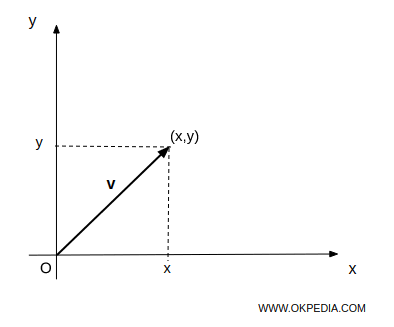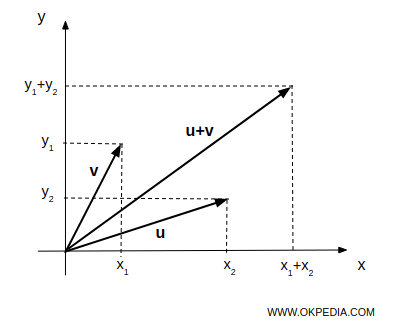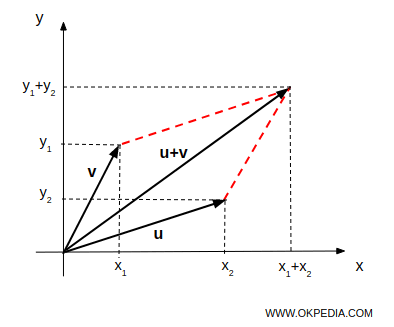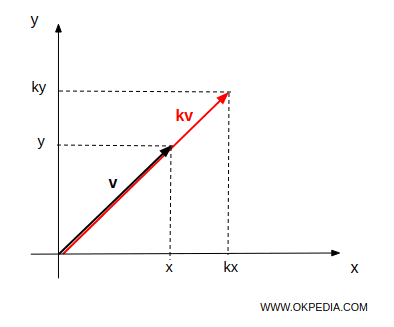# Vectors

Vectors are quantities characterized by both magnitude and direction. In two-dimensional or three-dimensional spaces, a vector is represented as an arrow with a specific length, originating from a starting point (O) and terminating at a point (x,y).A vector is essentially an ordered list of n values. These can be conceptualized as an n-tuple of real numbers. Each value is signified by a symbol with a subscript indicating its position in the list, for example, x1,x2, ..., xn

Vector notation typically employs a letter with an arrow above it, represented as

$$\vec{v} = ( x_1 \ , \ x_2 \ , ... , \ x_n )$$

Vectors are crucial for representing quantities that cannot be described by a single value. In physics, force is a practical example of a vector quantity.

Note. On Okpedia, vectors are differentiated from scalars by presenting them in bold. For instance, u, w, and v are vectors, while u, v, and w are scalars.

### Vector operations

Vectors can undergo various operations, including addition, subtraction, and scalar multiplication.

Each of these operations has specific rules and properties that dictate the resultant vector's magnitude and direction.

The primary operations involving vectors include:

If (x1,y1) and (x2,y2) are the endpoints of two vectors, u and v respectively, then (x1+x2,y1+y2) is the endpoint of the vector u+v.The sum of two vectors in the R²=RxR space can also be determined using the parallelogram method.• Scalar Multiplication
If (x, y) is the endpoint of vector v, then the product kv results in a vector with the endpoint (kx, ky).The product of a real number k and vector v is achieved by multiplying the magnitude of v by k. This product retains the same direction if k > 0, or reverses direction if k < 0.

www.okpedia.com - Okpedia - Contact email: info@okpedia.it - P.IVA - 09286581005 - Privacy Google - Disclaimer

Vectors

y